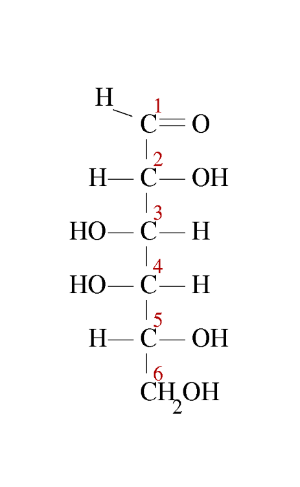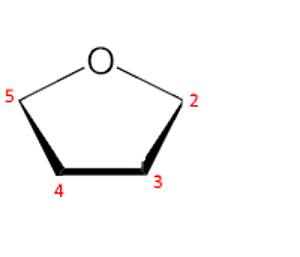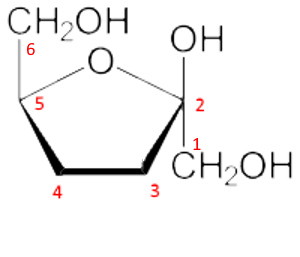﻿Search:

# Passage between linear representations and cyclic representations of D-aldoses and D-ketoses

## D - Aldoses

Example: The $\alpha$ - D - galactose

First step: The pyran cycle is oriented with oxygen behind and right. The carbons 1,2,3,4,5 are numbered from the right in a clockwise direction.Second step: We write the Fischer →       linear structure ($-CHO$ ​​in position 1 up!):Third step: The $-CH_2OH$ group, position 6, is attached in position 5 upwards:Fourth step: The new $-OH$ group in position 1, formed by the cyclization, is oriented downwards since one wishes to obtain $\alpha$ the form (for $\beta$ it would be orientated upwards):Fifth step: The $-OH$ groups in the remaining 2,3 and 4 positions are oriented downward or upward depending on whether they are to the right or left in the linear form:That's it!

## Ketoses

Example: The $\beta$ - D - fructose

First step: The furan ring is oriented with oxygen behind and in the center. The carbons 2,3,4,5 are numbered from the right in a clockwise direction.Second step: We write the Fischer →       linear structure ($-CO$ in position 2 up!):Third step: The $-CH_2OH$ group, position 6, is attached in position 5 upwards:Fourth step: The new $-OH$ group in position 2, formed by the cyclization, is oriented upward since we want to have the form $\beta$ (for $\alpha$ we would orient down). We don't forget the $CH_2OH$ in position 1.Fifth step: The $-OH$ groups in the remaining 3 and 4 positions are oriented downward or upward depending on whether they are to the right or left in the linear form:And there you go!